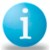# The Douglass Index Can Keep Good Data from Going to Waste

ByJim Douglass

In statistical process control, diligent technicians and auditors conduct detailed work to gather data for quality engineers, who then crunch the data into capability studies. Typically, the repeatability and reproducibility (R&R) rates for the data are first determined for the gauges used in the capability studies. The R&R studies are completed first to establish the credibility of the gauge prior to administering the capability study.

In a perfect world, firm R&R rates of 10 percent or lower would be recorded and the gauges would be applied in capability data collection. The lower R&R rate the better in a measuring system – as a rule of thumb, R&R rates less than 10 percent are ideal, and anything between 10 and 30 percent is acceptable. However, sometimes practitioners do not find a rate below 10 percent. For these cases, the author has developed the Douglass Index formula to connect a poor-to-marginal R&R of 10 to 30 percent to a marginal capability index (Cp) through a meaningful method of augmenting the former Cp to an improved Cp.

### Using the Index

The equation involved in the Douglass Index is a bit more complex than a simple correlation between two numbers. It is, however, a meaningful method of determining if the data is exhausted and needs to be redrawn or if it is valid and can remain as is. Accordingly, the purpose of the Douglass Index is to ensure that the existing data has been fully analyzed before the need arises to draft new data. The most functional way to apply the formula is as follows.

Layout the study results of a given machine or cell into a simple chart (see example in Table 1). For a broader scope, layout the study results of an entire department. After all, it is more useful to analyze a facility’s capability status departmentally. Be sure to include the corresponding R&R rates on the grid.

Table 1: Sample Study Results Matrix: North Annex

 Feature Studied Cp R&R % Cavity 1 1.98 8.1 Cavity 2 2.27 8.1 Gap 2.20 4.4 Center 2.39 6.3 Endplay 1.77 P Bezel A 2.21 3.8 Bezel B 2.30 3.8 Inner left 1.58 21.0 Inner right 1.78 21.0 Air gap 1.69 P Torque 4.45 P E-Dim 1.84 6.7 RPMs 1.52 28.0 Pressure stop 1.88 20.1 Rotate force 1.69 9.8 Piston swage 3.11 6.5 Socket stiffness 1.81 16.8 Voltage 2.02 2.1 P = Pass attribute

As can be expected, studying a measurable item using a gauge that garners a high bias due to sensitivity typically yields a marginal-to-poor R&R rate. But these marginal R&R rates become an integral factor in the Douglass Index equation. The team can analyze the study results grid to identify incapable processes or capability failures.

Handpicked Content:   Process Capability Calculations with Non-Normal Data

For example, say a capability study with a desired target of 1.67 or greater has yielded a failing Cp of 1.54. The team would compare this to the Douglass Index conversion table to learn if the R&R rate is 25 percent or less. If so, the index converts the former failing Cp of 1.54 to an improved 1.67 (Table 2). The index contains conversions to a Cp of 1.33 or, for more critical requirements, to a Cp of 1.67.

Table 2: Douglass Index Conversion Table

 R&R % Cp Old Cp New 29 1.24 1.33 26 1.26 1.33 22 1.28 1.33 19 1.29 1.33 16 1.30 1.33 14 1.31 1.33 11 1.32 1.33 29 1.50 1.67 28 1.51 1.67 27 1.52 1.67 26 1.53 1.67 25 1.54 1.67 24 1.55 1.67 23 1.56 1.67 22 1.57 1.67 21 1.57 1.67 20 1.58 1.67 19 1.59 1.67 18 1.60 1.67 17 1.61 1.67 16 1.61 1.67 15 1.62 1.67 14 1.63 1.67 13 1.63 1.67 12 1.64 1.67 11 1.64 1.67 10 1.65 1.67

### The Formula That Makes It Work

Before consulting the table, it may help for practitioners to understand the logic behind the Douglass Index. The equation subtracts the square root of the sum of the squares of the gauge inaccuracy from the actual part variation. This effectively removes that which makes the part variation falsely larger and further allows researchers to confirm if the data is acceptable.

For example, an unacceptable Cp of 1.58 equates to 1.67 in the index table if the R&R is 21 percent or less. Following this scenario, the formula to determine this conversion functions as follows:

• The process capability (reciprocal of the Cp x 100 percent) summates the sum of the squares (in other words, capability squared x R&R squared). The equipment variation is segregated by subtracting out the R&R factor.
• The reciprocal of the marginal Cp of 1.58 is 0.633 x 100 percent = 63.3 percent machine capability.
• The square of 63.3 is 4,006.
• Take the square of the poor R&R of 21 to get 441 and subtract 441 from 4006 = 3565.
• The square root of 3565 is 59.7÷100 = .597.
• Finally the reciprocal of .597 reaches the improved Cp of 1.67.
Handpicked Content:   How to Calculate Process Sigma

The full equation looks like this:

1÷1.58 = 0.633 x 100% = 63.3% 63.3²= 4,006 21²=441 4006-441=3565 √3565=59.7 ÷100 = .597 1÷.597 = 1.67 Cp

One note of caution: Avoid applying this formula in new program launches, new machine trial runs or in critical areas. The Douglass Index is better applied in scenarios of annual study cycles, existing machine qualifiers, supplier product monitoring and machine re-certifications. Further, it should also be noted the formula assumes that targeting is not an issue if Cp and Cpk are equal.

### Finding Value

The Douglass Index can be valuable in saving both time and money. Savings can be reaped each time a Cp is saved through the conversion tables, thus eliminating the need for further study or re-study. This allows diligent technicians and auditors to return to the shop to collect data on the next assignment and avoid rework one a previous one.